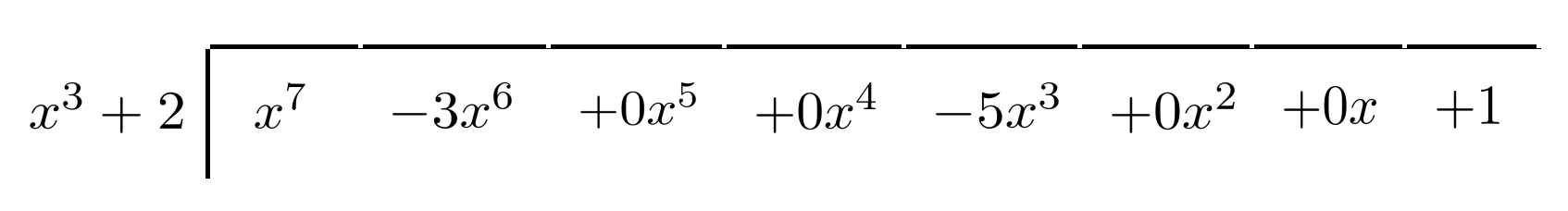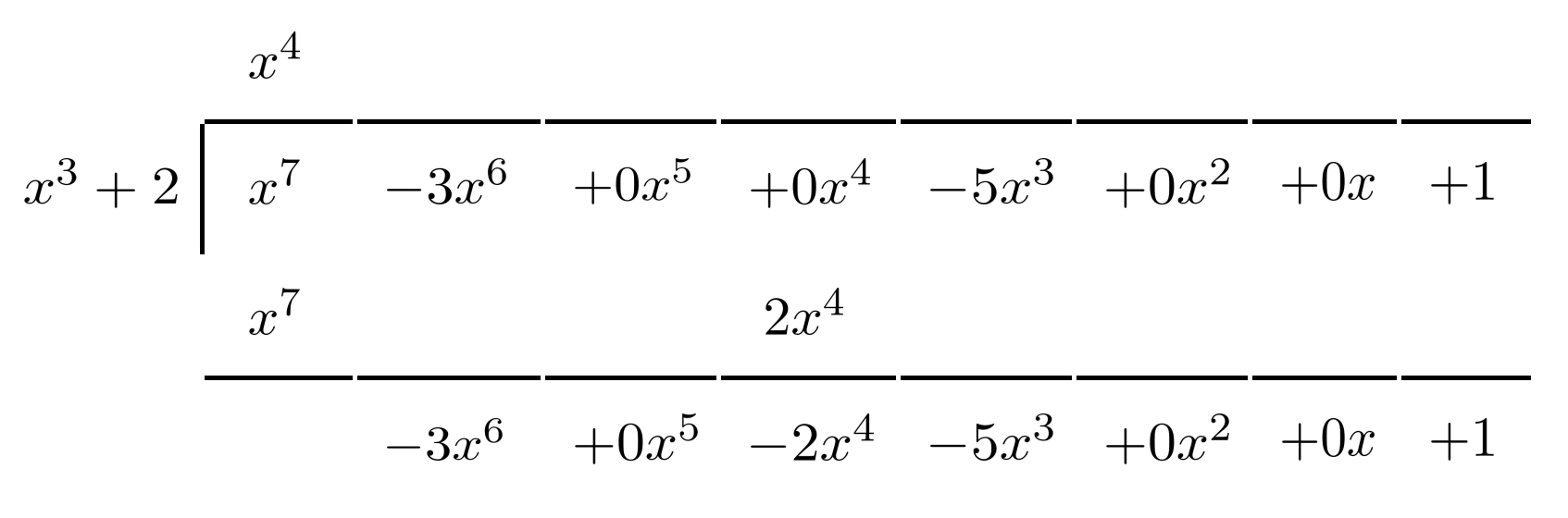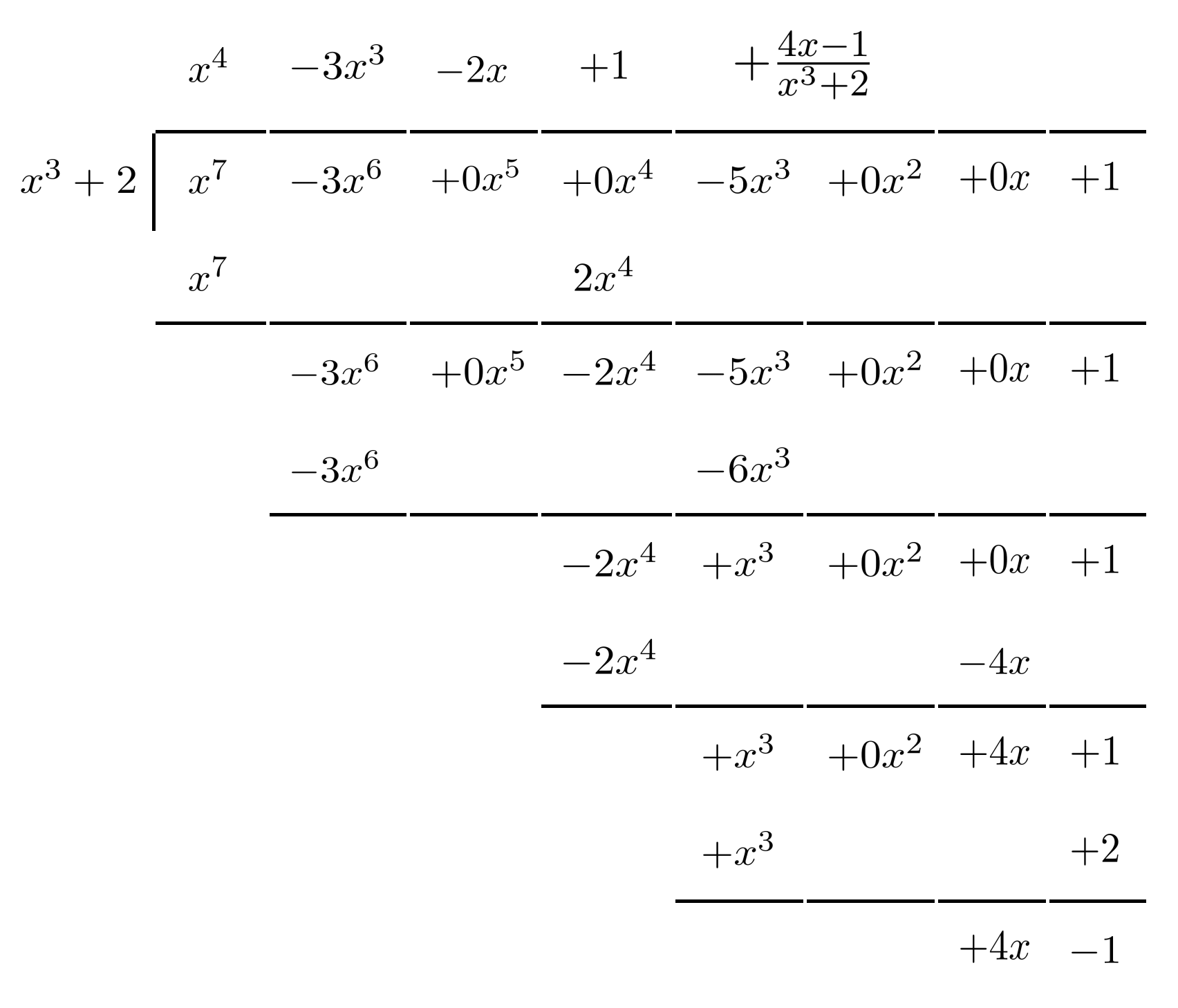# Polynomial Long Division

Polynomial long division works the same way as the long division algorithm that's familiar from simple arithmetic.

This post is part of a series.

A rational function is a fraction whose numerator and denominator are both polynomials. Rational functions are usually written in proper form, where the numerator is of a smaller degree than the denominator. (The degree of a polynomial is its highest exponent.)

## Methods for Converting to Proper Form

Sometimes, we can convert to proper form simply by splitting up the fraction.

\begin{align*} \frac{x+1}{x} \\ \frac{x}{x} + \frac{1}{x} \\ 1 + \frac{1}{x} \end{align*}

Other times, we can convert to proper form by factoring part of the numerator so that it cancels with the denominator.

\begin{align*} \frac{x^2+3x+5}{x+1} \\ \frac{(x^2+3x+2)+3}{x+1} \\ \frac{(x+1)(x+2)+3}{x+1} \\ \frac{(x+1)(x+2)}{x+1} + \frac{3}{x+1} \\ x+2+\frac{3}{x+1} \end{align*}

We can also use synthetic division, a fast algorithm for division of a linear factor that was introduced in the previous part on polynomials.

To divide $x^4+3x^3+2x-5$ by $x+2$, we set up a template with $-2$ (the zero of $x+2$) on the far left, and the coefficients of $x^4+3x^3+0x^2+2x-5$ along the top row.

After filling in an initial $0$, we repeatedly add down the columns, multiplying each result by $-2$ before placing it in the next column.The bottom row then tells us the coefficients and remainder in the proper form.

$\dfrac{x^4+3x^3+2x-5}{x+2} = x^3 + x^2 - 2x + 6 - \dfrac{17}{x+2}$

However, synthetic division only works with linear factors, so what do we do when a factor isn’t linear?

## Polynomial Long Division

When faced with more complicated rational functions, we can turn to polynomial long division, which works the same way as the long division algorithm that’s familiar from simple arithmetic.

To divide $x^7 - 3x^6 - 5x^3 + 1$ by $x^3+2$, we set up a template with $x^3+2$ on the outside and $x^7-3x^6-5x^3+1$ on the inside.

On the inside, we write out all coefficients, including those which are $0$ (and thus aren’t written in the condensed expression).We begin by multiplying the divisor $x^3+2$ by $x^4$ to yield $x^7+2x^4$, which cancels the interior $x^7$ term when we subtract.Then, we multiply $x^3+2$ by $-3x^3$ to yield $-3x^6-6x^3$, which cancels the next interior term $-3x^6$ when we subtract.

We repeat this process until the degree of the leftover terms is less than the degree of $x^3+2$, in which case the leftover terms become the remainder and appear as the numerator in the remaining fraction.The top row gives the result in proper form:

$\dfrac{x^7-3x^6-5x^3+1}{x^2+2} = x^4-3x^3-2x+1+ \dfrac{4x-1}{x^3+2}$

## Exercises

Find the proper form of each rational function. (You can view the solution by clicking on the problem.)

$1) \hspace{.5cm} r(x)=\dfrac{8x^5+7x^2+6}{4x^2}$
Solution:
$r(x)=2x^3+\dfrac{7}{4}+\dfrac{3}{2x^2}$

$2) \hspace{.5cm} r(x)=\dfrac{-9x^9+3x^7-2x^5+x^3+1}{3x^4}$
Solution:
$r(x)=-3x^5+x^3-\dfrac{2}{3}x + \dfrac{x^3+1}{3x^4}$

$3) \hspace{.5cm} r(x)=\dfrac{x^2+2x+1}{x+3}$
Solution:
$r(x)= x - 1 + \dfrac{4}{x+3}$

$4) \hspace{.5cm} r(x)=\dfrac{6x^2-5x-4}{2x^2+3x+1}$
Solution:
$r(x)= 3 - \dfrac{7}{x+1}$

$5) \hspace{.5cm} r(x)=\dfrac{2x^5+3x^4+x^2-x+5}{x+2}$
Solution:
$r(x)= 2x^4 -x^3 + 2x^2 - 3x + 5 - \dfrac{5}{x+2}$

$6) \hspace{.5cm} r(x)=\dfrac{-4x^4+x^2+4x-4}{2x-1}$
Solution:
$r(x)= - 2x^3 - x^2 + 2 - \dfrac{2}{2x-1}$

$7) \hspace{.5cm} r(x)=\dfrac{3x^4+20x^2+2x+22}{x^2+5}$
Solution:
$r(x)= 3x^2 + 5 + \dfrac{2x-3}{x^2+5}$

$8) \hspace{.5cm} r(x)=\dfrac{-3x^7+x^6-8x^5+5x^4-5x^3+3x^2+2}{x^3+2x-1}$
Solution:
$r(x)= -3x^4 + x^3 - 2x^2 + \dfrac{x^2+2}{x^3+2x-1}$

$9) \hspace{.5cm} r(x)=\dfrac{x^7-2x^6-x^5+2x^3-x^2-6x-3}{x^5+x+1}$
Solution:
$r(x)= x^2-2x-1 + \dfrac{x^3-3x-2}{x^5+x+1}$

$10) \hspace{.5cm} r(x)=\dfrac{6x^8+4x^7+3x^5+2x^4-9x^3-6x^2+1}{3x^3+2x^2}$
Solution:
$r(x)= 2x^5 + x^2 - 3 + \dfrac{1}{3x^3+2x^2}$

$11) \hspace{.5cm} r(x)=\dfrac{x^{13}-4x^{10}+6x^9-17x^6+8x^4-15x^3-2x+14}{x^6-4x^3+2}$
Solution:
$r(x)= x^7 + 6x^3 - 2x + 7 + \dfrac{x^3+2x}{x^6-4x^3+2}$

$12) \hspace{.5cm} r(x)=\dfrac{-18x^{12}+2x^{11}+25x^9+4x^8-x^7+3x^6-23x^5+22x^4-x^3}{9x^4-x^3+x+1}$
Solution:
$r(x)= -2x^8 + 3x^5 + x^4 - 3x + 2 + \dfrac{x^3 + 3x^2 + x-2}{9x^4 - x^3 + x + 1}$

This post is part of a series.

Tags: